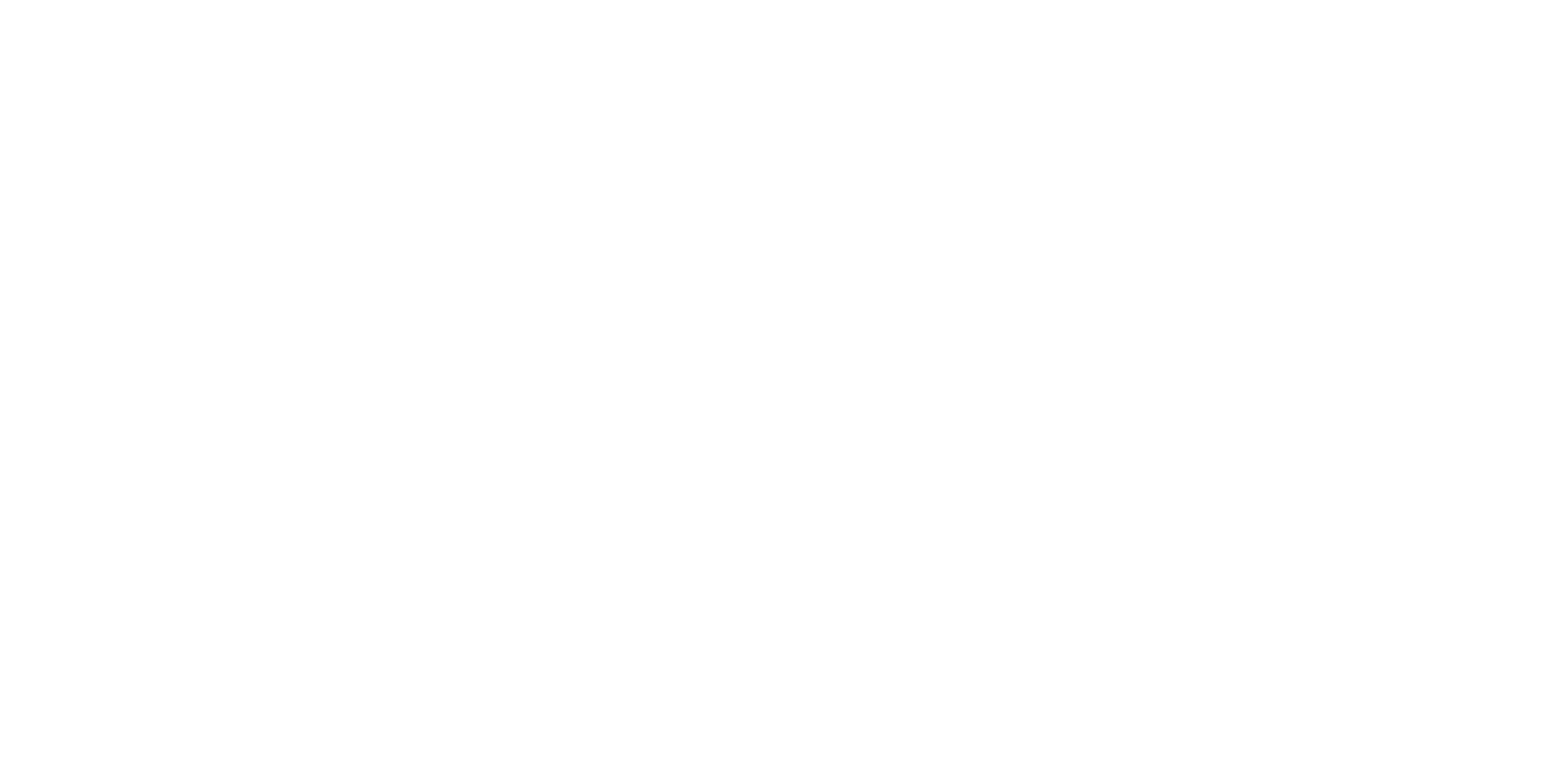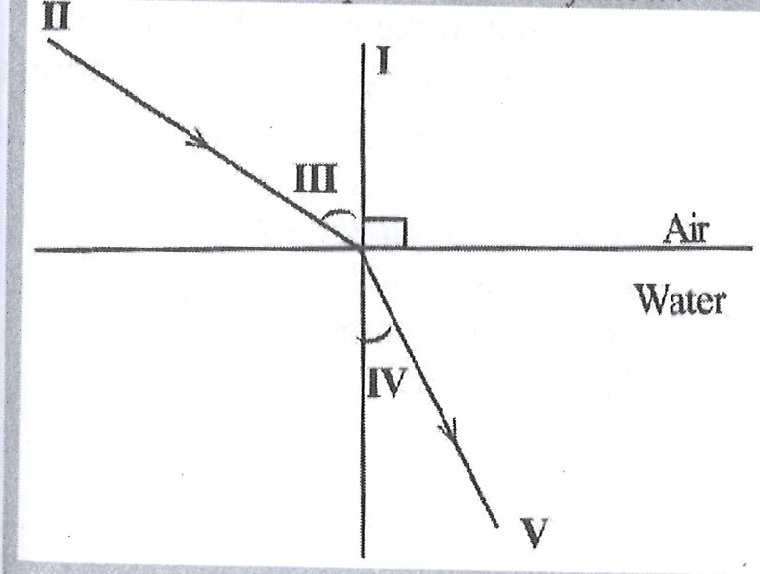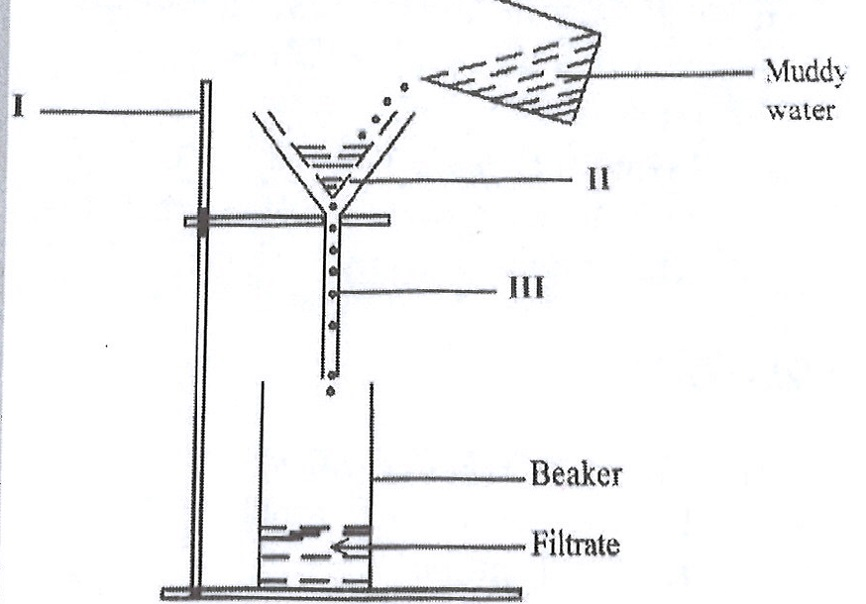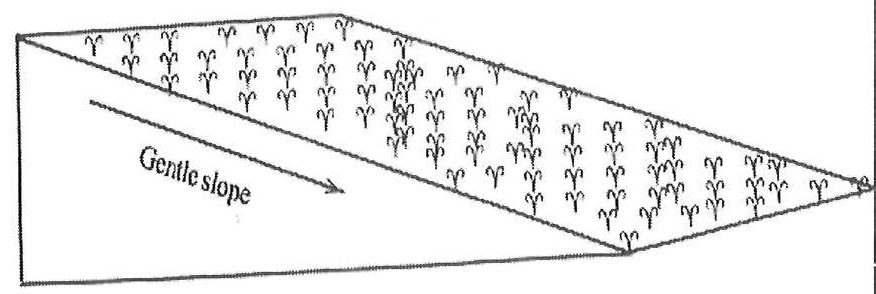Kuulchat

Online Education Resource Portal, its all freeBECE SCIENCE 2017

#### OBJECTIVE TEST

1.

The instrument used to measure relative humidity is the

A.

hydrometer.

B.

hygrometer.

C.

rain gauge.

D.

wind vane.

2.

The S.I unit for density is

A.

ms-1

B.

ms-2

C.

kg m-3

D.

m3kg-1

3.

When light travels from glass to air, its speed is

A.

increased.

B.

reduced.

C.

halved.

D.

unchanged.

4.

When a piece of iron rod is brought near a permanent magnet for a while it becomes magnetised by

A.

stroking method.

B.

electrical method.

C.

friction.

D.

induction.

5.

A rheostat is used in an electric circuit in order to

A.

increase electric current only.

B.

decrease electric current only.

C.

keep electric current constant.

D.

increase and decrease electric current.

6.

Which of the following statements about an opaque object is correct? An opaque object

A.

allows light to pass through it partially.

B.

allows light to pass through it fully.

C.

D.

7.

One of the characteristics of a soil is that

A.

its particles are tightly packed.

B.

it assumes the shape of the container it occupies.

C.

it has no fixed volume.

D.

its particle can easily be separated.

8.

The instrument used to measure current in an electric circuit is the

A.

ammeter.

B.

photometer.

C.

thermometer.

D.

voltmeter.

9.

The bottom of a pool of water appears closer to the surface than it is. Which of the following properties accounts for this observation?

A.

Reflection.

B.

Transparency water

C.

Refraction

D.

Rectilinear propagation

10.

An example of a source of energy which is non-renewable is

A.

petroleum.

B.

solar.

C.

tide.

D.

wind.

11.

Which of the following apparatus is not required in the process of distillation in the laboratory?

A.

Condenser

B.

Evaporating dish

C.

Bunsen burner

D.

12.

The number of oxygen atoms present in three molecules of carbon dioxide is

A.

3.

B.

4.

C.

5.

D.

6.

13.

The chemical formula Cl2 represents two

A.

atoms of chlorine.

B.

neutrons of chlorine.

C.

ions of chlorine.

D.

molecules of chlorine.

14.

Which of the following substances can rust?

A.

Aluminium

B.

Bauxite

C.

Copper

D.

Steel

15.

Energy stored in food is referred to as

A.

chemical energy.

B.

heat energy.

C.

solar energy.

D.

kinetic energy.

16.

Which of the following substances is a mixture?

A.

Water

B.

Sodium chloride

C.

Sodium chloride solution

D.

Iron filing

17.

The mole is the S.I unit of

A.

amount of substance.

B.

luminous intensity.

C.

temperature.

D.

mass of substance.

18.

An atom has a proton number of 17 and neutron number 18. Determine the number of electrons in the third shell of the atom.

A.

1

B.

3

C.

5

D.

7

19.

An example of mixtures that can be separated by the method of filtration is

A.

sugar in water.

B.

sand in water.

C.

oil in water.

D.

ink in water.

20.

Which of the following liquids would turn blue litmus paper red?

A.

Vinegar

B.

Water

C.

Ammonia solution

D.

Bicarbonate solution

21.

Which of the following particles constitute matter?

I. Atoms

II. Molecules

III. Ions

A.

I only

B.

II only

C.

II and III only

D.

I, II and III

22.

An example of inheritable characteristics in humans is

A.

shape of nose.

B.

knowledge.

C.

ability to speak different languages.

D.

handwriting.

23.

Which of the following statements about aerobic respiration is correct?

A.

The by-product are carbon dioxide and water.

B.

Little amount of energy is produced.

C.

Oxygen is not required.

D.

The by-products are alcohol and carbon dioxide.

24.

Sperms produced by the testes in humans are temporarily stored in the

A.

epididymis.

B.

prostate gland.

C.

seminal vesicles.

D.

sperm duct.

25.

Which of the following changes normally occur during old age in humans?

A.

Grey hair starts appearing

B.

C.

D.

Attraction to the the opposite sex

26.

One of the characteristics of wind pollinated flowers is that they

A.

are large and conspicuous.

B.

are usually scented.

C.

have nectar.

D.

have feathery stigma.

27.

The testes are normally located outside the body of the male because

A.

the temperature of the body is too high for effective sperm production.

B.

they cannot increase in size when it is in the body.

C.

they can absorb moisture from the atmosphere.

D.

they occupies too much space.

28.

The organelle which occupies the largest portion of a plant cell is the

A.

chloroplast.

B.

mitochondrion.

C.

nucleus.

D.

vacuole.

29.

Movements of the ribs is brought about by the

A.

pleural cavity.

B.

intercostal muscle.

C.

larynx.

D.

trachea.

30.

Fertilisation in humans usually occurs in the

A.

ovary.

B.

uterus.

C.

vagina.

D.

fallopian tube.

31.

Which of the following processes is an example of osmosis?

A.

Spreading of perfume in a room.

B.

Absorption of food nutrients into the small intestines.

C.

Spreading of potassium permanganate in water.

D.

Absorption of water into the root hairs.

32.

The part of a living cell which is semi-permeable is

A.

cytoplasm.

B.

nucleus.

C.

protoplasm.

D.

cell membrane.

33.

Which of the following statements about soil profile is/are correct? It helps the farmer to determine

I. soil fertility.

II. water-holding capacity of the soil.

III. depth of the soil.

A.

I only

B.

I and II only

C.

II and III only

D.

I, II and III

34.

Which of the following practices is not a principle of crop rotation?

A.

Legumes should be added to the rotation programme.

B.

Deep rooted crops should be followed by shallow rooted crops.

C.

Crops that are closely related should follow each other.

D.

Fallow period should be allowed during the time of rotation.

35.

The relative proportion of sand, silt and clay in a given sample of soil soil

A.

profile.

B.

porosity.

C.

structure.

D.

texture.

36.

The first factors to be considered when deciding to cultivate a particular vegetable is

A.

cultural practice.

B.

selection of land.

C.

harvesting.

D.

method of propagation

37.

Which of the following should be considered when slecting a site for vegetable crop production?

I. Nearness to the market

II. Topography of the land

III. Nearness to the sea

A.

I and II only

B.

I and III only

C.

II and III only

D.

I, II and III

38.

Which of the following activities are cultural practices in the cultivation of cabbage?

I. Prunning

II. Weeding

III. Watering

A.

I and II only

B.

I and III only

C.

II and III only

D.

I, II and III only

39.

An example of a vegetable crop is

A.

cocoa.

B.

cotton.

C.

potato.

D.

tomato.

40.

The majority charge carriers is a p-type semi-conductor are

A.

electrons.

B.

holes.

C.

neutrons.

D.

protons.

#### THEORY QUESTIONS

1.

(a) The diagram below is an illustration of a longitudinal section of a canine tooth in humans.

Study the diagram carefully and answer the questions that follow.(i) Name each of the parts labelled I, II, III and IV.

(ii) What is the function of each of the parts labelled I and III?

(iii) Which of the labelled parts could be affected by tooth decay?

(iv) State three ways by which tooth decay may be prevented.

(b) The diagram below is an illustration of a scientific phenomenom which occurs in nature.

Study the diagram carefully and answer the questions that follow.(i) What phenomenom does the diagram illustrate?

(ii) Identify each of the parts labelled I, II, III, IV and V.

(iii) Explain why an object at the bottom of a pond appears closer to the surface than it actually is.

(c) The diagram below is an illustration of an experiment performed to separate the components of muddy water.

Study the diagram carefully and answer the questions that follow.(i) Name each of the parts labelled I, II, and III.

(ii) State the function of the part labelled II.

(iii) Name the substance obtained as the filtrate.

(iv) State three physical properties of the filtrate.

(v) Name two other materials that could be used in place of the part labelled II.

(d) The diagram below is an illustrates a farmland on a sloppy area.

Study the diagram carefully and answer the questions that follow.(i) What process is likely to occur on the farmland when it rains heavily?

(ii) State two farming practices that can also lead to the process mentioned in (i).

(iii) List four farming practices that could be used to control the process mentioned in (i).

(iv) Mention three soil resources that would be depleted from the farmland when it rains heavily.

Show Solution
2.

(a) An atom Y has atomic number 12. It loses two electrons in order to be stable.

(i) State the proton number of the atom before it loses electrons.

(ii) State the electron number of the atom:

(α) before it loses electrons.

(β) after losing electrons.

(iii) Name the type of ion formed by the atom when it loses two electrons.

(b) Name four farming system in crop production.

(c) (i) What is dispersal of seed?

(ii) State two characteristics of seeds dispersed by wind.

(d) Explain the term forward bias of a p-n junction diode.

Show Solution
3.

(a) (i) What is an acid?

(ii) Give two difference between an acid and a base, in terms of taste and feel.

(b) (i) Define pressure.

(ii) A force of 200N is exerted on an area of 50m2. Calculate the pressure exerted by the force

(c) Explain the following terms as associated with living organisms.

(i) unicellullar;

(ii) multicellullar.

(d) Give two reasons why soil air is important.

Show Solution
4.

(a) (i) Explain the following terms as applied to machines:

(α) work input;

(β) work output.

(ii) State one factor that limits work output for a given work input in a simple machine.

(b) (i) What is chloroplast?

(ii) Differentiate between aerobic respiration and anaerobic respiration.

(c) (i) State the colour change that would occur when blue litmus paper is dipped onto a solution of:

(α) vinegar;

(β) wood ash.

(ii) Name the products formed when hydrochloric acid reacts with sodium hydroxide.

(d) List two benefits of vegetables to humans.

Show Solution
5.

(a) (i) Differentiate between egestion and digestion in nutrition.

(ii) What is the end-product of digestion?

(b) Give one example of a chemical compound used in:

(i) medicine;

(ii) agriculture;

(iii) industry.

(c) (i) Define the term soil profile.

(ii) State two ways in which soil profile is important in crop production.

(d) (i) State two steps used by scientists in doing their work.

(ii) Give two subjects that may be considered as applied sciences.

Show Solution
6.

(a) (i) What is an alloy?

(ii) State two causes of corrosion of metals.

(b) (i) What is a planet?

(ii) Name two planets between the Sun and the Earth.

(c) State four functions of the circulatory system in humans.

(d) (i) Define the term crop rotation.

(ii) Give one example of a chemical method of controlling pests on crop farms.

Show Solution